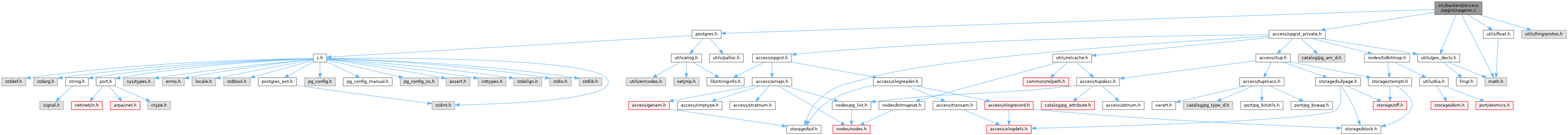PostgreSQL Source Code  git master
spgproc.c File Reference
`#include "postgres.h"`
`#include <math.h>`
`#include "access/spgist_private.h"`
`#include "utils/builtins.h"`
`#include "utils/float.h"`
`#include "utils/geo_decls.h"`
Include dependency graph for spgproc.c:Go to the source code of this file.

## Macros

#define point_point_distance(p1, p2)

## Functions

static double point_box_distance (Point *point, BOX *box)

double * spg_key_orderbys_distances (Datum key, bool isLeaf, ScanKey orderbys, int norderbys)

BOXbox_copy (BOX *orig)

## ◆ point_point_distance

 #define point_point_distance ( p1, p2 )
Value:
#define DirectFunctionCall2(func, arg1, arg2)
Definition: fmgr.h:644
static Datum PointPGetDatum(const Point *X)
Definition: geo_decls.h:181
Datum point_distance(PG_FUNCTION_ARGS)
Definition: geo_ops.c:1993
static float8 DatumGetFloat8(Datum X)
Definition: postgres.h:494

Definition at line 25 of file spgproc.c.

## ◆ box_copy()

 BOX* box_copy ( BOX * orig )

Definition at line 82 of file spgproc.c.

83 {
84  BOX *result = palloc(sizeof(BOX));
85
86  *result = *orig;
87  return result;
88 }
void * palloc(Size size)
Definition: mcxt.c:1226
Definition: geo_decls.h:141

References palloc().

## ◆ point_box_distance()

 static double point_box_distance ( Point * point, BOX * box )
static

Definition at line 31 of file spgproc.c.

32 {
33  double dx,
34  dy;
35
36  if (isnan(point->x) || isnan(box->low.x) ||
37  isnan(point->y) || isnan(box->low.y))
38  return get_float8_nan();
39
40  if (point->x < box->low.x)
41  dx = box->low.x - point->x;
42  else if (point->x > box->high.x)
43  dx = point->x - box->high.x;
44  else
45  dx = 0.0;
46
47  if (point->y < box->low.y)
48  dy = box->low.y - point->y;
49  else if (point->y > box->high.y)
50  dy = point->y - box->high.y;
51  else
52  dy = 0.0;
53
54  return HYPOT(dx, dy);
55 }
static float8 get_float8_nan(void)
Definition: float.h:123
#define HYPOT(A, B)
Definition: geo_decls.h:91
Point low
Definition: geo_decls.h:143
Point high
Definition: geo_decls.h:142
float8 y
Definition: geo_decls.h:99
float8 x
Definition: geo_decls.h:98

References get_float8_nan(), BOX::high, HYPOT, BOX::low, Point::x, and Point::y.

Referenced by spg_key_orderbys_distances().

## ◆ spg_key_orderbys_distances()

 double* spg_key_orderbys_distances ( Datum key, bool isLeaf, ScanKey orderbys, int norderbys )

Definition at line 63 of file spgproc.c.

65 {
66  int sk_num;
67  double *distances = (double *) palloc(norderbys * sizeof(double)),
68  *distance = distances;
69
70  for (sk_num = 0; sk_num < norderbys; ++sk_num, ++orderbys, ++distance)
71  {
72  Point *point = DatumGetPointP(orderbys->sk_argument);
73
74  *distance = isLeaf ? point_point_distance(point, DatumGetPointP(key))
76  }
77
78  return distances;
79 }
static Point * DatumGetPointP(Datum X)
Definition: geo_decls.h:176
static BOX * DatumGetBoxP(Datum X)
Definition: geo_decls.h:234
#define point_point_distance(p1, p2)
Definition: spgproc.c:25
static double point_box_distance(Point *point, BOX *box)
Definition: spgproc.c:31